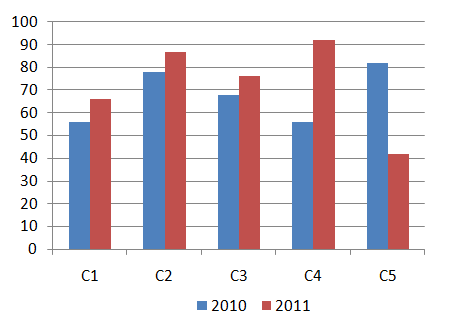# RPF 10 Jan 2019

Instructions

For the following questions answer them individually

Question 111

# A bag contains different types of old coins in the ratio 11:13:12 whose values are 3 rupees, 4 rupees and 5 rupees respectively. If the total value of the coins is Rs.725, find the number of coins of 3 rupees coin.Question 112

# The difference between the interest earned on the same amount invested under compound interest and simple interest at same rate of interest for 2 years is Rs.28. If the rate of interest is 4% p.a. then find the amount invested.(in Rs.)Question 113

# The amount doubles itself under Compound interest in 3 years. In how many years will it become 8 times of it?Question 114

# The salary ratio of Ram, Raj and Rio is 5:7:9, if Rio is getting Rs.280 more than Ram. What is the salary of Raj?(in Rs.)Question 115

# Study the following bar graph and answer the question that follows:Sales of books (in thousands) from 5 branches (C1,C2,C3,C4&C5) of a publishing company for the two successive years 2010 and 2011. What is the average sales of all the branches (in thousands) for the year 2010?Question 116

# Time taken by Binu and Caro to cover a distance of 1000 km are in the ratio 17:14. Find the ratio of their speeds.Question 117

# Sachin shared 250 gifts among 4 kids. The share of the first kid, twice the share of second kid, thrice the share of third kid and four times the share of fourth kid are all equal. Find the number of gifts received by 2nd kid.Question 118

# When a number divided by 36 it leaves a remainder 7. What will be the remainder, when the same number divided by 9.Question 119

# Darmik obtained 71 marks out of 80 in French, 81 marks out of 100 in English, 51 out of 70 in Spanish and 31 out of 50 in Japanese. What was the overall percentage obtained by him?Question 120

# A shopkeeper sells a product at the rate of Rs.1089 and earns a profit of 12.5%. Find the amount which is equal to half of the cost price of the product.OR